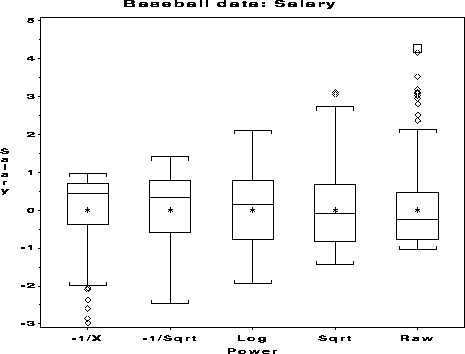symbox Boxplots for transformations to symmetry symbox

# SAS Macro Programs: symbox

\$Version: 1.2 (07 Apr 2005)
Michael Friendly
York University

## The symbox macro (get symbox.sas)

### Boxplots for transformations to symmetry

Various graphical displays for diagnosing symmetry of a distribution are available in the SYMPLOT macro. SYMBOX takes a more direct approach. The variable is first transformed to each of a series of selected powers, then each transform is standardized to mean=0, std=1.

The results are then displayed side-by-side in boxplots, permiting a visual assessment of which power makes the distribution reasonably symmetric.

Missing and non-positive observations are excluded.

### Parameters

DATA=_LAST_
Name of the input data to be analyzed.
VAR=
Name of the variable to be plotted. Only one variable may be specified.
POWERS=0 .5 1
A list of two or more powers for the VAR= variable to be transformed. A power of 0 is taken to mean 'log10(VAR)'. Negative powers are taken to mean '-1 / VAR**|power|'.
OUT=SYMOUT
Name of the output data set
NAME=SYMBOX
The name assigned to the graph(s) in the graphic catalog.

### Example

This example examines the distribution of the variable SALARY in the Baseball dataset.
```%include data(baseball);

title h=1.6 "Baseball data: Salary";
%symbox(data=baseball, var=Salary, powers =-1 -.5 0 .5 1 );
```### See also

boxcox Power transformations by Box-Cox method
boxplot Box-and-whisker plots
stars Diagnostic plots for transformations to symmetry